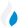•Trade Pi
•Trade
•Exchange
•Trade Pi
•Trade
•Exchange
\$1.12T
Total marketcap
\$78.5B
Total volume
40.94%
BTC dominance
•Trade Pi
•Trade
•Exchange

# PUNDIX to FX Exchange Rate - 1 Pundi X in Function X

2.2724099179437
• BTC 0.000019
• ETH 0.0003
Vol [24h]
\$52,533.48

## pundix to fx converter

Exchange Pair Price 24h volume
Fx Swap PUNDIX/FX \$0.4494 \$52.51K

## PUNDIX/FX Exchange Rate Overview

Name Ticker Price % 24h 24h high 24h low 24h volume
Pundi X pundix \$0.459 1.0565% \$0.465 \$0.4316 \$4.25M
Function X fx \$0.1985 1.0281% \$0.2 \$0.1927 \$1.15M

Selling 1 PUNDIX you get 2.2724099179437 Function X fx.

Pundi X Mar 31, 2021 had the highest price, at that time trading at its all-time high of \$10.07.

672 days have passed since then, and now the price is 4.56% of the maximum.

Based on the table data, the PUNDIX vs FX exchange volume is \$52,533.48.

Using the calculator/converter on this page, you can make the necessary calculations with a pair of Pundi X/Function X.

## Q&A

### What is the current PUNDIX to FX exchange rate?

Right now, the PUNDIX/FX exchange rate is 2.2724099179437.

### What has been the Pundi X to Function X trading volume in the last 24 hours?

Relying on the table data, the Pundi X to Function X exchange volume is \$52,533.48.

### How can I calculate the amount of FX? / How do I convert my Pundi X to Function X?

You can calculate/convert FX from Pundi X to Function X converter. Also, you can select other currencies from the drop-down list.

## PUNDIX to FX Сonversion Table

PUNDIX FX
1 PUNDIX = 2.2 FX
2 PUNDIX = 4.5 FX
4 PUNDIX = 9.08 FX
7 PUNDIX = 15.9 FX
20 PUNDIX = 45.4 FX
30 PUNDIX = 68.1 FX
40 PUNDIX = 90.8 FX
200 PUNDIX = 454.4 FX
300 PUNDIX = 681.7 FX
3000 PUNDIX = 6,817.2 FX
30000 PUNDIX = 68,172.2 FX
300000 PUNDIX = 681,722.9 FX
3000000 PUNDIX = 6,817,229.7 FX